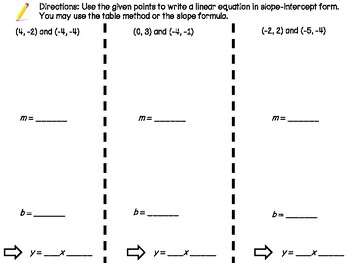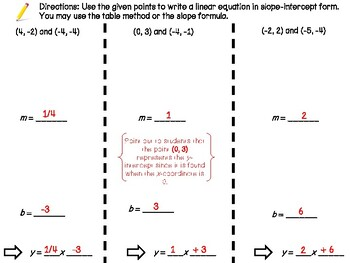# Slope Intercept Given 2 PointsSubject
Resource Type
File Type

PDF

(238 KB|5 pages)
Product Rating
Standards
• Product Description
• StandardsNEW

This foldable is designed for use with interactive notebooks but may be used in isolation. The activity provides guided notes and practice problems that will guide students through the process of writing a linear equation in slope-intercept form when given to points.

This product includes the foldable (which will need to be xeroxed front to back) as well as the corresponding ANSWER KEY!

• Guided notes on slope-intercept given 2 points
• 2 methods of determining slope
• Solving for a given variable
• Identifying slope and y-intercept of an equation
• Rate of change
Describe qualitatively the functional relationship between two quantities by analyzing a graph (e.g., where the function is increasing or decreasing, linear or nonlinear). Sketch a graph that exhibits the qualitative features of a function that has been described verbally.
Construct a function to model a linear relationship between two quantities. Determine the rate of change and initial value of the function from a description of a relationship or from two (𝘹, 𝘺) values, including reading these from a table or from a graph. Interpret the rate of change and initial value of a linear function in terms of the situation it models, and in terms of its graph or a table of values.
Interpret the equation 𝘺 = 𝘮𝘹 + 𝘣 as defining a linear function, whose graph is a straight line; give examples of functions that are not linear. For example, the function 𝘈 = 𝑠² giving the area of a square as a function of its side length is not linear because its graph contains the points (1,1), (2,4) and (3,9), which are not on a straight line.
Compare properties of two functions each represented in a different way (algebraically, graphically, numerically in tables, or by verbal descriptions). For example, given a linear function represented by a table of values and a linear function represented by an algebraic expression, determine which function has the greater rate of change.
Understand that a function is a rule that assigns to each input exactly one output. The graph of a function is the set of ordered pairs consisting of an input and the corresponding output.
Total Pages
5 pages
Included
Teaching Duration
45 minutes
Report this Resource to TpT
Reported resources will be reviewed by our team. Report this resource to let us know if this resource violates TpT’s content guidelines.